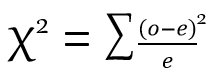# How to Calculate a Chi Square: Formula & Example

An error occurred trying to load this video.

Try refreshing the page, or contact customer support.

Coming up next: What is the Center in a Data Set? - Definition & Options

### You're on a roll. Keep up the good work!

Replay
Your next lesson will play in 10 seconds
• 0:04 Chi Square Formula
• 1:25 Calculating Chi Square
• 3:32 Lesson Summary

Want to watch this again later?

Timeline
Autoplay
Autoplay
Speed

#### Recommended Lessons and Courses for You

Lesson Transcript
Instructor: Mia Primas

Mia has taught math and science and has a Master's Degree in Secondary Teaching.

In this lesson, you will learn the formula and method for calculating chi square. You can then take a brief quiz to see what you learned. You will learn how to interpret the value of chi square in a separate lesson.

## Chi Square Formula

Chi square is a method used in statistics that calculates the difference between observed and expected data values. It is used to determine how closely actual data fit expected data. The value of chi square helps us to answer the question, 'is the difference in expected and observed data statistically significant?' A small chi square value tells us that any differences in actual and expected data are due to chance, so the data is not statistically significant. A large value tells us the data is statistically significant and there is something causing the differences in data. From there, a statistician may explore factors that may be responsible for the differences.Chi is a Greek symbol that looks like the letter x as you can see in the 'chi square formula' image on screen now. To calculate chi square, we take the square of the difference between the observed (o) and expected (e) values and divide it by the expected value. Depending on the number of categories of data, we may end up with two or more values. Chi square is the sum of those values. It's a common mistake for students to take the square root, as you would if you were solving for chi. However, the value that we are looking for is chi square, so we do not need to take the square root.

Once we have the value of chi square, we would use a chi square table to determine whether the data is statistically significant.

## Calculating Chi Square

A poll was taken of 100 college freshmen to determine whether chocolate or vanilla was the preferred ice cream flavor. It was expected that 25% would prefer chocolate and 75% would prefer vanilla. Let's look at the results of the survey. Instead of 25% of the students polled preferring chocolate, it turns out that 39% preferred it, and instead of 75% of the students polled preferring vanilla, it turned out that 61% preferred it.

Chocolate Vanilla
Expected 25 75
Observed 39 61

Because we have two categories, chocolate and vanilla, we will need to calculate two values and take their sum. This will give us the value of chi square. The expected value gets substituted for e in the equation, and the observed value gets substituted for o.

To unlock this lesson you must be a Study.com Member.

### Register to view this lesson

Are you a student or a teacher?

### Unlock Your Education

#### See for yourself why 30 million people use Study.com

##### Become a Study.com member and start learning now.
Back
What teachers are saying about Study.com

### Earning College Credit

Did you know… We have over 160 college courses that prepare you to earn credit by exam that is accepted by over 1,500 colleges and universities. You can test out of the first two years of college and save thousands off your degree. Anyone can earn credit-by-exam regardless of age or education level.